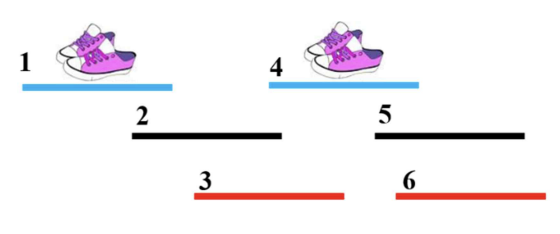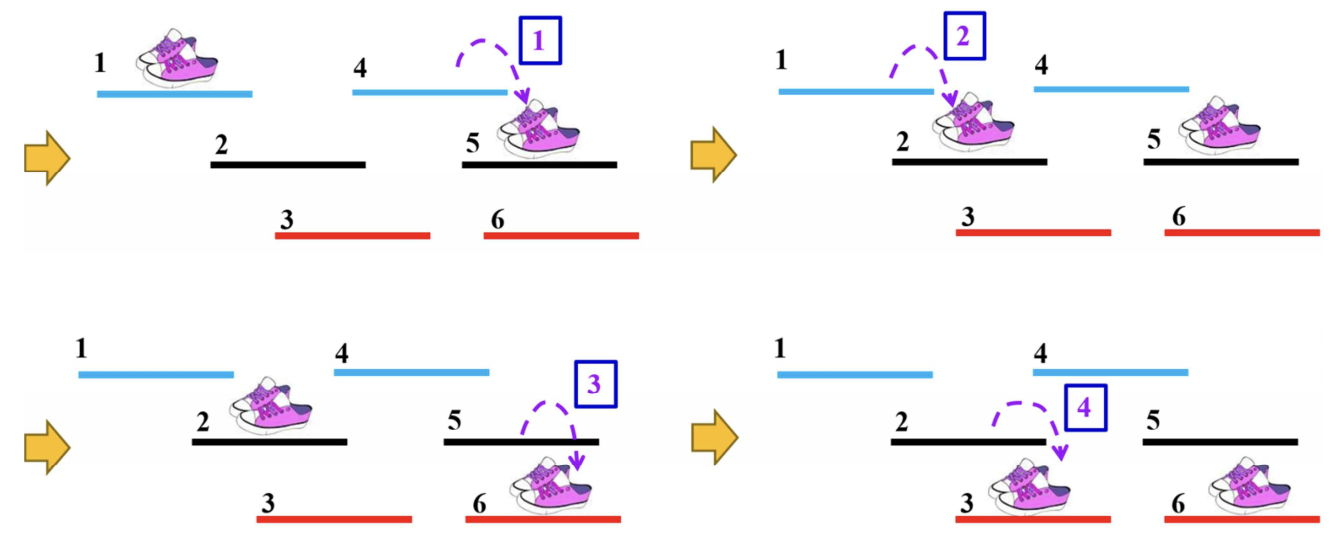시간 제한 메모리 제한 제출 정답 맞은 사람 정답 비율
2 초 (추가 시간 없음) 512 MB 7 5 4 100.000%

## 문제

Korean idol groups are famous in the world for dynamic and elaborate group dances, called Kalgunmu. A Korean idol group has m members. One day the members practice their choreography in a stage. Suppose that the stage is simplified as a line. There are n closed intervals of identical length on the stage. Here we shall specify seven rules which the members should follow in every moment of choreography as follows:

• R1) Each member should stand on one of the intervals.
• R2) No two or more members can stand on the same interval.
• R3) Two intervals on which arbitrary two members stand respectively cannot intersect.

Note that two closed intervals [a, b] and [c, d] with ac intersect if cb.

The choreography consists of several steps with the following rules:

• R4) At any step, only one member can move from her/his current interval to another interval. In other words, two or more members cannot move simultaneously at any step.
• R5) When changing the interval on which she/he stands at some step, a member has to move into one

of the intervals that her/his current interval intersects.

Note that a member cannot move into the interval I if I intersects an interval on which another member stands, according to the rule R3.

• R6) The set of intervals on which the members stand in the start of choreography should be S.
• R7) The set of intervals on which the members stand in the end of choreography should be E.

Note that the sets S and E are given as in the input.

According to the rules of the choreography, the size of S (or E) is m and the intervals in S (or E) do not intersect each other. Also each member shall move from an interval s in S to an interval e in E through the choreography. We are interested in the minimum number of steps to complete the choreography satisfying the above rules R1 to R7.

For example, in Figure E. 1, there are six intervals on a stage, which are denoted by numbers from 1 to 6. The blue intervals 1 and 4 are intervals on which two members stand in the start of choreography and the red intervals 3 and 6 are ones in the end.Figure E. 1 Initial configuration S of the choreography on a stageFigure E. 2 Four steps of choreography

At the first step, the member standing on the interval 4 moves into the interval 5. Notice that the member on the interval 1 cannot move into the interval 2 at the first step, because the interval 2 intersects the interval 4 that another member stands on. As in Figure E. 2, two members can move into the red intervals in four steps. But if the member on the interval 4 moves into the interval 6 at the first step, then the choreography would be completed in three steps, which is minimum.

Given m members, n intervals, and two sets S and E for the choreography, write a program to find a choreography from S to E with a minimum number of steps, and output all such steps.

## 입력

Your program is to read from standard input. The input starts with a line containing two integers, m and n (1 ≤ mn ≤ 5,000), where m is the number of members and n is the number of intervals on the stage. The intervals are numbered from 1 to n such that the left endpoint of interval i is strictly less than the left one of interval i + 1. The second line contains an integer r, the length of intervals (1 ≤ r ≤ 10,000). The third line contains a sequence of sorted n integers in ascending order where the i-th number is the left endpoint of the interval i, where these numbers are between 0 and 109. The following line contains a sequence of sorted m integers to denote the intervals belonging to the set S in ascending order. The last line contains a sequence of sorted m integers to denote the intervals belonging to the set E in ascending order.

## 출력

Your program is to write to standard output. The first line should contain the minimum number MIN of steps to complete the choreography. In the following MIN lines, the i-th line contains two integers a and b, where a member moves from the interval a to the interval b at the step i of the choreography with the minimum number of steps MIN. If there are one or more solutions, then print arbitrary one. If there is no solution, then print −1.

## 예제 입력 1

2 6
6
1 6 8 11 15 16
1 4
3 6


## 예제 출력 1

3
4 6
1 2
2 3


## 예제 입력 2

2 6
6
1 6 8 11 15 16
1 4
1 4


## 예제 출력 2

0


## 예제 입력 3

2 6
3
1 6 8 11 15 16
1 4
3 6


## 예제 출력 3

-1


## 출처

• 데이터를 만든 사람: jh05013
• 스페셜 저지를 만든 사람: jh05013